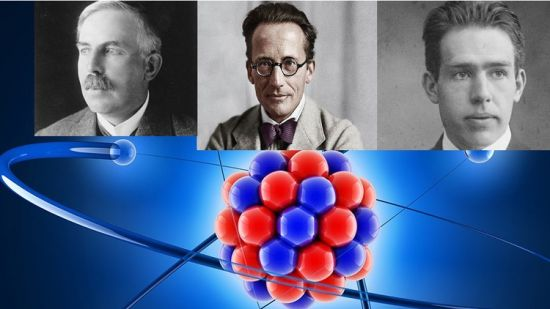﻿ Derivation of the energy spectrum of the hydrogen atom » GFXhome

» » Derivation of the energy spectrum of the hydrogen atom
Information of news
20-06-2021, 09:28

### Derivation of the energy spectrum of the hydrogen atom

Category: TutorialsMP4 | Video: h264, 1280x720 | Audio: AAC, 44.1 KHz, 2 Ch
Genre: eLearning | Language: English + srt | Duration: 10 lectures (2h 1m) | Size: 1 GB

Quantum physics and Schrodinger equation applied to the Hydrogen atom
What you'll learn:
How to derive the discrete energy spectrum of a hydrogen-like atom from the Schrodinger equation
How to use the method of separation of variables to solve the Schrodinger equation

Requirements
Calculus, Multivariable Calculus (especially: derivatives, the Laplacian, spherical coordinates)
Concept of potential energy, Coulomb interaction
Some familiarity with the Schrodinger equation (not how to solve it, but what it is, what the wave function and Hamiltonian are)
complex exponentials
Some familiarity with ordinary differential equations

Description
In this course the discrete energy spectrum of hydrogen-like atoms is derived from the Schrodinger equation. In the following, the history of this important discovery is contextualized.

In the early 20th century, Ernest Rutherford performed some experiments that established that atoms consisted of negatively charged electrons surrounding a small, dense, positively charged nucleus. From the experimental data, Rutherford was led to consider a planetary model of the atom, the Rutherford model of 1911. This had electrons orbiting a nucleus, but involved a technical difficulty: the laws of classical mechanics predict that the electron will release electromagnetic radiation while orbiting a nucleus. Because the electron would lose energy, it would rapidly collapse into the nucleus in a very minuscule amount of time (of the order of picoseconds). This model of the atom is disastrous because it predicts that all atoms are unstable. However, late 19th-century experiments had shown that atoms will only emit light (that is, electromagnetic radiation) at certain discrete frequencies.

To overcome the problems of Rutherford's atom, in 1913 Niels Bohr put forth a new model which would correctly describe the energy levels of hydrogen-like atoms.

In 1925, a new kind of mechanics was proposed, quantum mechanics, in which Bohr's model of electrons traveling in quantized orbits was extended into a more accurate model of electron motion. The new theory was proposed by Werner Heisenberg. Another form of the same theory, wave mechanics, was discovered by the famous Austrian physicist Erwin Schrödinger independently, and by different reasoning. Schrödinger employed de Broglie's matter waves, but sought wave solutions of a three-dimensional wave equation describing electrons that were constrained to move about the nucleus of a hydrogen-like atom, by being trapped by the potential of the positive nuclear charge.

Who this course is for
Students who want to understand the mathematics behind the energy spectrum of the hydrogen atomSite BBcode/HTML Code:
Dear visitor, you went to the site as unregistered user.